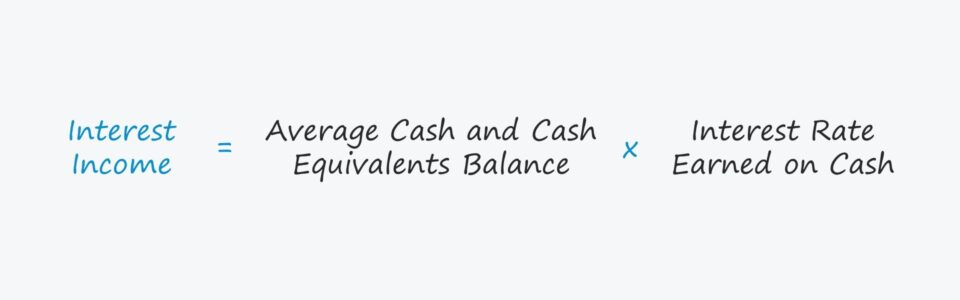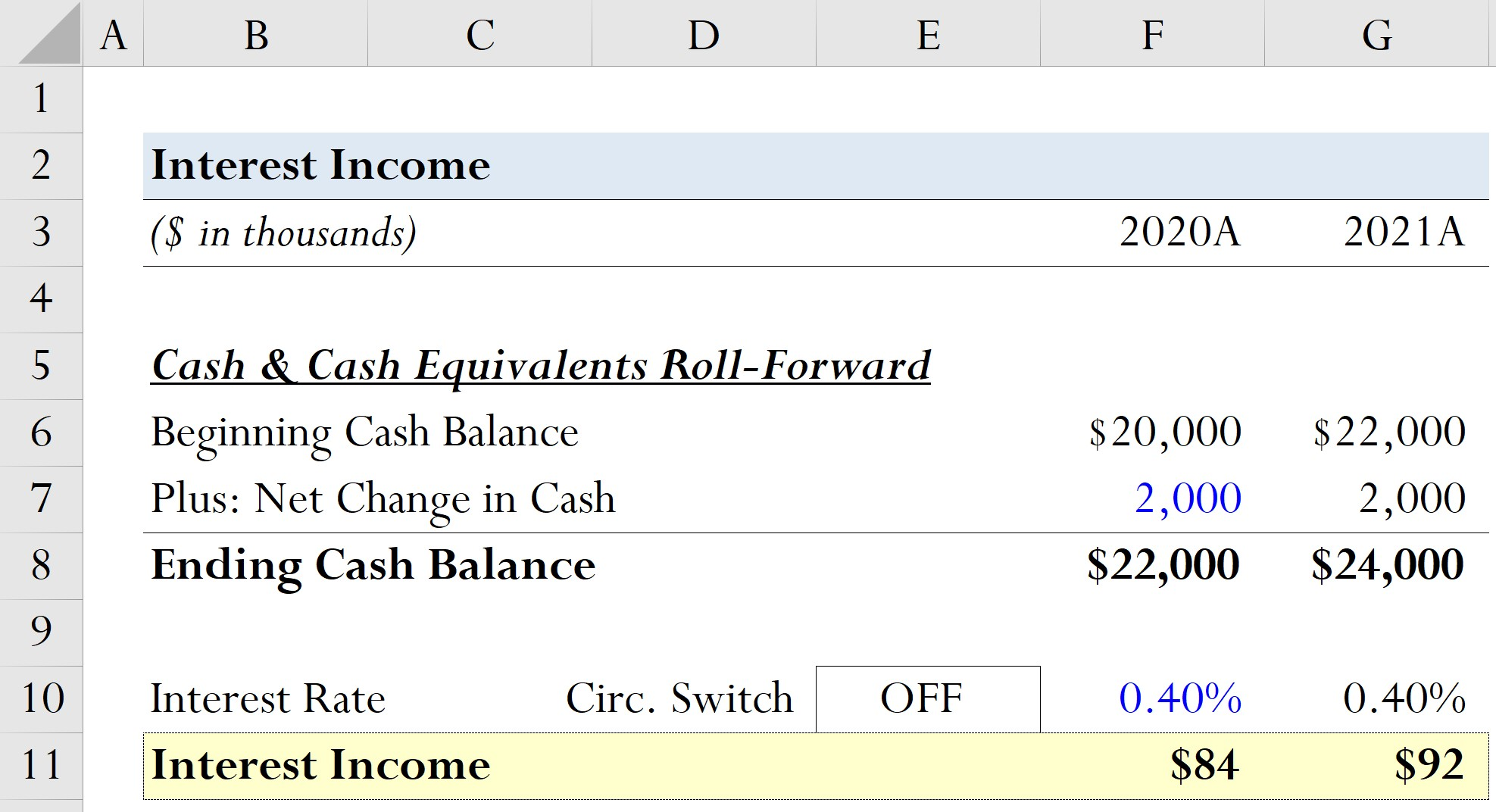Welcome to Wall Street Prep! Use code at checkout for 15% off.# Interest Income

Guide to Understanding Interest Income## Interest Income: Accounting Definition

Companies retain cash and cash equivalents on their balance sheet to ensure they have sufficient liquidity to meet short-term financing and working capital needs.

Cash not reinvested into operations is frequently invested into interest-yielding accounts such as the following:

These sorts of short-term investments typically carry low yields, but it still enables the company to earn a return and offset losses from having “idle” cash.

For most companies – excluding financial institutions such as commercial banks – interest is reported in the non-operating items section of the income statement.

The interest earned is not considered a non-financial company’s core part of operations, i.e. it is not integral to the company’s normal course of business.

## How to Calculate Interest Income (Step-by-Step)

A company’s interest income is determined by its projected cash balances and an interest rate assumption.

With that said, the forecasted interest income can only be computed once the balance sheet and cash flow statement are complete.

Calculating a company’s interest income is a two-step process:

1. Calculate Average Cash and Cash Equivalents Balance (Between Beginning and End of Period)
2. Multiply Cash and Cash Equivalents Balance by the Interest Rate Earned (%)

In Excel, the standard method used to forecast either type of interest – interest income and interest expense – creates a “circularity” within a financial model, which we’ll discuss how to circumvent later in our modeling tutorial.

## Interest Income Formula

The formula to calculate the interest income is as follows.

Interest Income = Average Cash and Cash Equivalents Balance Interest Rate Earned on Cash (%)

## Interest Income vs. Interest Expense

Companies often consolidate interest expense with interest income into a single line item called “Interest Expense, net” on their income statement.

In such cases, it is worth the time to locate the individual amounts broken out separately, so that each item can be referenced and projected in the forecast.

Like its counterpart, interest expense, interest income is modeled when building out the debt schedule of a financial model. Hence, interest is considered one of the “finishing touches” of a 3-statement model.

On the income statement, interest income and interest expense are often presented together, but there is a clear distinction between the two items:

• Interest Income: Interest income is the cash “earned” by a company from depositing its funds into low-risk investments such as marketable securities, government bonds and certificate of deposits (CDs).
• Interest Expense: In contrast, interest expense is the cost of borrowing from lenders and represent the payments “incurred” by a company as part of funding its day-to-day operations (e.g. working capital, capital expenditures).

## Interest Income Calculator – Excel Template

We’ll now move to a modeling exercise, which you can access by filling out the form below.Submitting...

## Step 1. Balance Sheet Assumptions

Suppose a company’s beginning cash balance was \$20 million in 2020. Further, we’ll assume the net change in cash – i.e. the total movement of cash in the specified period – is an increase of \$2 million across both periods.

2020A Cash Balances

• Beginning Cash Balance = \$20 million
• Plus: Net Change in Cash = \$2 million
• Ending Cash Balance = \$22 million

2021A Cash Balances

• Beginning Cash Balance = \$22 million
• Plus: Net Change in Cash = \$2 million
• Ending Cash Balance = \$24 million

## Step 2. Interest Income Formula in Excel (Circularity)

Moreover, the interest rate earned on cash for both periods will be set at 0.40%.

• Interest Rate (%) = 0.40%

The formula for calculating the interest income in Excel is as follows:

=IF(Circ=0,0,Interest Rate*AVERAGE (Beginning Cash Balance, Ending Cash Balance))

While not necessary for our simple exercise, setting up a circularity switch is crucial in a properly integrated 3-statement model.

• Circ = 0: If the cell named “Circ” is set to zero, the formula calculates the interest as zero.
• Circ = 1: Conversely, the formula could also be set so that if the circularity switch is turned on, only the beginning cash balance is used for the calculation.

## Step 3. Interest Income Calculation Example

The interest income comes out as \$84k in 2020, which increases to \$92k in 2021 due to the positive rise in the cash balance on a year-over-year (YoY) basis.

• Interest Income, 2020A = 0.40% * Average (\$20 million, \$22 million) = \$84,000
• Interest Income, 2021A = 0.40% * Average (\$22 million, \$24 million) = \$92,000Step-by-Step Online Course

### Everything You Need To Master Financial Modeling

Enroll in The Premium Package: Learn Financial Statement Modeling, DCF, M&A, LBO and Comps. The same training program used at top investment banks.